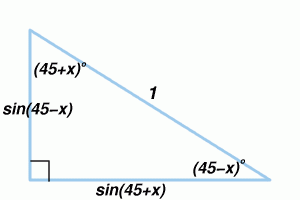Draw a triangle with angles x, (45 + x) and (45 – x) degrees.

What does Pythagoras Theorem tell you about these angles?

Use this information to find this sum of squares of sines:

$$\sin^2 1 + \sin^2 2 + \cdots + \sin^2 359 + \sin^2 360$$

See the AIMSSEC Notes for Teachers.

This problem is adapted from the NRICH task Degree Ceremony with permission of the University of Cambridge. All rights reserved.